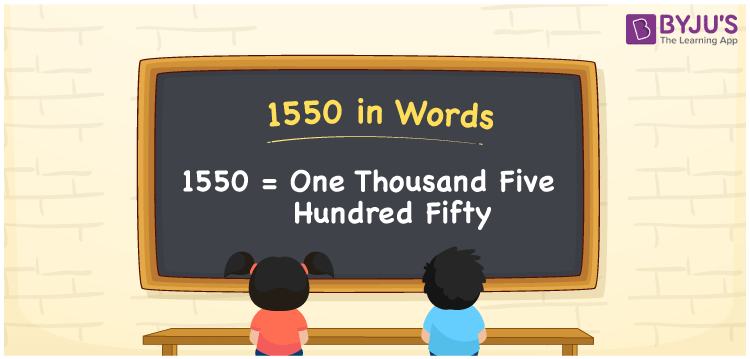# 1550 in Words

The number name of 1550 is One Thousand Five Hundred Fifty. 1550 is a cardinal number that can be converted into words using the place value system. If the cost of the book is Rs. 1550, it can be written as “The cost of the book is Rs. One thousand five hundred fifty”. In this article, we will discuss how to write 1550 in words with a complete explanation.

 1550 in Words: One Thousand Five Hundred Fifty One Thousand Five Hundred Fifty in Numerical Form: 1550

## 1550 in English Words

The number 1550 in English words is as follows:## How to Write 1550 in Words?

The number 1550 in words can be written using the place value of the number. Below is the place value table that represents the place value of 1550.

 Thousands Hundreds Tens Ones 1 5 5 0

Following is the expanded form of the number 1550.

= 1 × Thousand + 5 × Hundred + 5 × Ten + 0 × One

= 1 × 1000 + 5 × 100 + 5 × 10

= 1000 + 500 + 50

= 1550

= One Thousand Five Hundred Fifty

Hence, 1550 in words is One Thousand Five Hundred Fifty.

1550 in words – One Thousand Five Hundred Fifty

Is 1550 an odd number? – No

Is 1550 an even number? – Yes

Is 1550 a perfect square number? – No

Is 1550 a perfect cube number? – No

Is 1550 a prime number? – No

Is 1550 a composite number? – Yes

## Frequently Asked Questions (FAQs) on 1550 in Words

Q1

### How to write 1550 in words?

1550 in words is One Thousand Five Hundred Fifty.

Q2

### Simplify 1500 + 50, and express it in words.

Simplifying 1500 + 50, we get 1550. Hence, 1550 in words is One Thousand Five Hundred Fifty.

Q3

### How to write one thousand five hundred fifty in numbers?

One thousand five hundred fifty in numbers is 1550.

Q4

### How do you write 1550 in Words on a Cheque?

On a cheque, we generally write 1550 in words as One Thousand Five Hundred Fifty.
Q5

### Is 1550 an Even Number?

Yes.1550 is an Even Number.